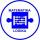# Sequence 2

Write the first 5 members of an arithmetic sequence a11=-14, d=-1

a1 =  -4
a2 =  -5
a3 =  -6
a4 =  -7
a5 =  -8

### Step-by-step explanation:

${a}_{1}=-14-\left(11-1\right)\cdot \left(-1\right)=-4$
${a}_{2}={a}_{1}-1=-5$
${a}_{3}={a}_{2}-1=-6$
${a}_{4}={a}_{3}-1=-7$
${a}_{5}={a}_{4}-1=-8$Did you find an error or inaccuracy? Feel free to write us. Thank you!## Related math problems and questions:

• SequenceWrite the first 6 members of this sequence: a1 = 5 a2 = 7 an+2 = an+1 +2 an
• SequenceWrite the first 7 members of an arithmetic sequence: a1=-3, d=6.
• Sequence 3Write the first 5 members of an arithmetic sequence: a4=-35, a11=-105.
• Sequence - 5 membersWrite first five members of the sequence ?
• Five membersWrite first 5 members geometric sequence and determine whether it is increasing or decreasing: a1 = 3 q = -2
• Sequence 11What is the nth term of this sequence 1, 1/2, 1/3, 1/4, 1/5?
• AS - sequenceWhat are the first ten members of the sequence if a11=22, d=2.
• SequenceBetween numbers 1 and 53 insert n members of the arithmetic sequence that its sum is 702.
• AP - simpleFind the first ten members of the sequence if a11 = 132, d = 3.
• Finite arithmetic sequenceHow many numbers should be inserted between the numbers 1 and 25 so that all numbers create a finite arithmetic sequence and that the sum of all members of this group is 117?
• Geometric sequence 3In geometric sequence is a8 = 312500; a11= 39062500; sn=1953124. Calculate the first item a1, quotient q, and n - number of members by their sum s_n.
• GP membersThe geometric sequence has 10 members. The last two members are 2 and -1. Which member is -1/16?
• Geometric sequence 4It is given geometric sequence a3 = 7 and a12 = 3. Calculate s23 (= sum of the first 23 members of the sequence).
• AS sequenceIn an arithmetic sequence is given the difference d = -3 and a71 = 455. a) Determine the value of a62 b) Determine the sum of 71 members.
• Sum of membersWhat is the sum of the first two members of the aritmetic progression if d = -4.3 and a3 = 7.5?
• Geometric sequence 5About members of geometric sequence we know: ? ? Calculate a1 (first member) and q (common ratio or q-coefficient)
• SequenceIn the arithmetic sequence is a1=-1, d=4. Which member is equal to the number 203?